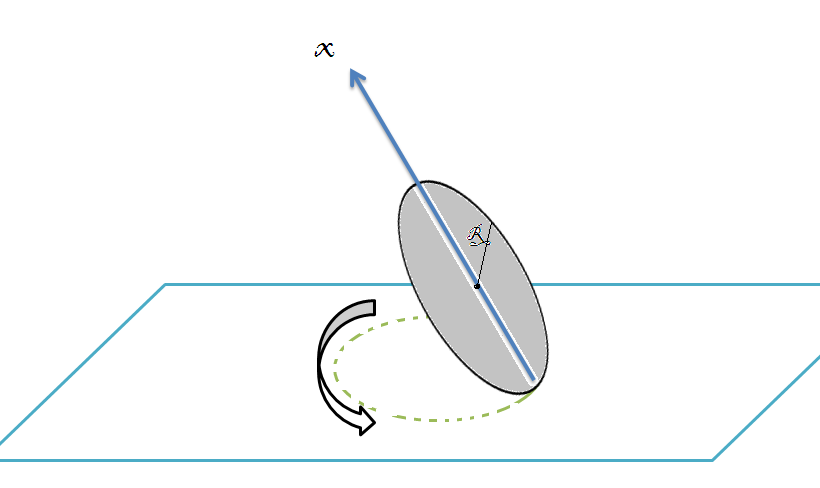# Wobbly WobbleIf you spin a coin around a vertical diameter on a table, it will slowly lose energy and begin a wobbling motion. The angle between the coin and the table will gradually decrease, and eventually, it will come to rest.

Assume that this process is slow, and consider the motion when the coin makes an angle $\theta$ with the table. You may assume that the center of mass is essentially motionless. Let $R$ be the radius of the coin, and $\Omega$ be the frequency at which the contact point on the table traces out its circle. Assume that the coin rolls without slipping.

The angular velocity of the coin is $\omega=c\Omega \sin\theta \hat{x}$, where $\hat{x}$ points upward along the coin, directly away from the contact point. If $\omega=p \sqrt{\dfrac{kg}{R(\sin\theta)^{k}}}$ and $p,k,c$ are integers, then, find $p \times k \times c$,.

×

Problem Loading...

Note Loading...

Set Loading...